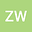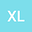Generalized hyperbolic mean curvature flow in Minkowski space $R^{1,1}$
••This paper concerns the generalized hyperbolic mean curvature flow for spacelike curves in Minkowski $R^{1,1}$. Base on the derived quasilinear hyperbolic system, we investigate the formation of singularities in the motion of these curves. In particular, under the generalized hyperbolic mean curvature flow, we prove that the motion of periodic spacelike curves with small variation on one period and small initial velocity blows up in finite time. Some blowup results have been obtained and the estimates on the life-span of the solutions are given.